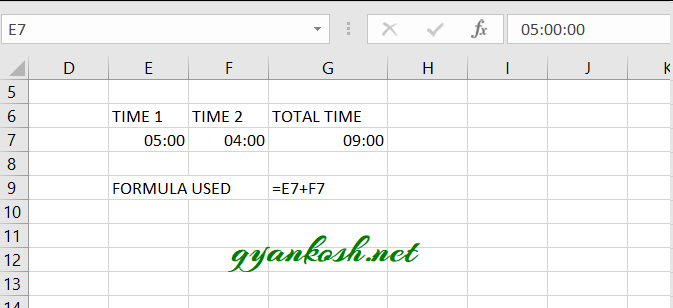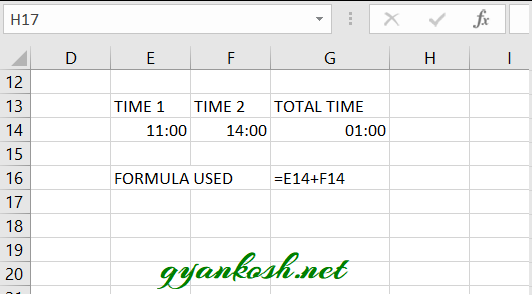PROVIDING ACCURATE, RELIABLE AND STRAIGHT TO THE POINT KNOWLEDGE ABOUT DIFFERENT IT TOOLS EXPLAINED WITH PRACTICAL EXAMPLES

# HOW TO ADD TIME IN EXCEL

## INTRODUCTION

DATES and TIME , if you are new to Excel and you have already tried using those format, I am pretty sure that you must have felt panicky at some sort of time.
It is quite obvious because when we don’t know the exact working of any system or process, we try to use it just by hit and trial method. It works sometimes and sometimes not.
Here we are talking about Dates and Time in Excel. These are the tricky formats which we need frequently in our reports or charts.
Many times, we need to do operations on them. We need to put them in the conditions to trigger some event which makes it very important for us to learn the exact procedures to perform a task concerned with the dates and time.
In this article we would learn different tricks and methods to handle and manipulate Dates and Time formats so that they don’t mess up with our reports.
One of such situations is when we need to ADD THE TIME IN EXCEL.
BEFORE READING THIS ARTICLE , IT IS REQUESTED TO VISIT THE PART I WHICH DISCUSSES THE CONCEPT OF THE DATE AND TIME IN EXCEL FOR BETTER UNDERSTANDING.CLICK HERE TO VISIT.

Adding the time is again a very important utility in excel but if we don’t know the proper way to handle it, it can create problem for us.

Let us try to find out the different ways to find out the sum of time in Excel.

WHENEVER WE TRY TO PERFORM OPERATIONS ON DATE OR TIME, ALWAYS REMEMBER THAT THEY ARE NUMBERS AND TRY TO PERFORM CALCULATIONS ON NUMBERS FIRST.

We can simply add the hours as they are the number for example
5:00 hrs+ 6 hrs=11 hrs.
But some cases create confusion.
Let us discuss the different cases one by one.

### STEPS TO ADD THE TIME IN EXCEL

•  Enter the given times in different cells. For the example we take the two cell and put different cases in them.
• Enter the following formula in the third cell.
• =Cell 1 containing time+ Cell 2 containing time +…. so on.
• The result will appear in the result cell.[ Where we have put the formula].

### EXAMPLE 1: TIME 1=5 HRS TIME 2=4 HRS.In the above picture we can see that the simple total has been performed and that is fine.

### EXAMPLE 2:

TIME 1=11:00 HRS
TIME 2=14:00 HRS
Let us try to sum them up.Look at the picture above. We tried to sum 11 hrs and 14 hrs but the answer is 1 hr instead of 25 hrs.

So is it wrong??
It is not wrong. If we remind the excel system we know that upto 24 hrs it counts for one day. So as soon as the sum exceeds the 24 hrs it counts it as one day and one hr.
If we convert the cell to the LONG DATE [ which will show both date and time], we would find that 1:00 hr is of the next date.
But yes! we have the solution.
We’ll change the format so that we get the solution as pure hours and not dates.
Revising the same example
Just in place of Summing up the times, we will put it in the TEXT FUNCTION.

### EXAMPLE 3:

Let us put all the complications in this example and try our formula if it still works or not.

 TIME 1 11:53 TIME 2 12:59 TIME 3 14:59 TIME 4 06:30 TIME 5 10:15 TIME 6 16:15 TIME 7 32:20:00 TIME 8 64:40:00

We use the following function for this.

=TEXT(SUM(F34:F41),”[HH]:MM”)

Where F34 to F41 has all the time stored which is summed up.

When it is summed up, it’ll change the date after every 24 hrs but as we want the result in hours only we use the TEXT FORMULA and give the format as [HH]:MM which will give the total hours and minutes as answer.

• ## WHAT IS EXCEL, HOW EXCEL WORKS, WRITE FORMULA IN EXCEL, EXAMPLE OF USING FORMULA IN EXCEL, ANIMATED EXAMLPE OF USING FORMULA IN EXCEL

LEARN

• EXCEL
• JAVASCRIPT
• MORE TO COME…

Encourage us by following at …

If you have any queries or suggestions or any problem which you would like us to solve for you, kindly mail at

[email protected]